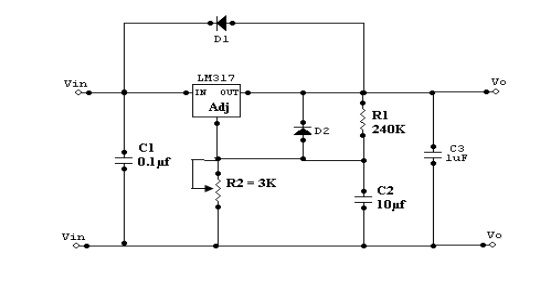Home | | Linear Integrated Circuits | Classifications of IC voltage regulators

# Classifications of IC voltage regulators

Fixed & Adjustable output Voltage Regulators are known as Linear Regulator.

Classifications of IC voltage regulators:·           Fixed & Adjustable output Voltage Regulators are known as Linear Regulator.

·           A series pass transistor is used and it operates always in its active region. Switching Regulator:

1.        Series Pass Transistor acts as a switch.

2.        The amount of power dissipation in it decreases considerably.

3.        Power saving result is higher efficiency compared to that of linear.

1.        Adjustable output voltage from 1.2v to 57 v

2.        Output current 0.10 to 1.5 A

3.        Better load & line regulation

·           LM317 series adjustable 3 terminal positive voltage regulator, the three terminals are Vin, Vout& adjustment (ADJ).

·           LM317 requires only 2 external resistors to set the output voltage.

·           LM317 produces a voltage of 1.25v between its output & adjustment terminals. This voltage is called as Vref.

·           Vref (Reference Voltage) is a constant, hence current I1 flows through R1 will also be constant. Because resistor R1 sets current I1. It is called ―current set‖ or ―program resistor‖.

·            Resistor R2 is called as ―Output set‖ resistors, hence current through this resistor is the sum of I1

·           LM317 is designed in such as that Iadj is very small & constant with changes in line voltage & load current.

·           The output voltage Vo is, Vo=R1I1+(I1+Iadj)R2 ------------- (1)

Where I1= Vref/R1

Vo =(Vref/R1)R1 + Vref/R1 + Iadj R2

= Vref + (Vref/R1)R2 + Iadj R2

Vo = Vref [1 + R2/R1] + Iadj R2-------------       (2)

R1 = Current (I1) set resistor

R2 = output (Vo) set resistor

Vref = 1.25v which is a constant voltage between output and ADJ terminals.

·           Current Iadj is very small. Therefore the second term in (2) can be neglected.

·           Thus the final expression for the output voltage is given by

Vo= 1.25v[1 + R2/R1]--------------       (3)

Eqn (3) indicates that we can vary the output voltage by varying the resistance R2. The value of R1 is normally kept constant at 240 ohms for all practical applications.

Practical Regulator using LM317:·           If LM317 is far away from the input power supply, then 0.1μf disc type or 1μf tantalum capacitor should be used at the input of LM317.

·           The output capacitor Co is optional. Co should be in the range of 1 to 1000μf.

·           The adjustment terminal is bypassed with a capacitor C2 this will improve the ripple rejection ratio as high as 80 dB is obtainable at any output level.

·           When the filter capacitor is used, it is necessary to use the protective diodes.

·           These diodes do not allow the capacitor C2 to discharge through the low current point of the regulator.

·           These diodes are required only for high output voltages (above 25v) & for higher values of output capacitance 25μf and above.

Study Material, Lecturing Notes, Assignment, Reference, Wiki description explanation, brief detail
Linear Integrated Ciruits : Application of ICs : Classifications of IC voltage regulators |